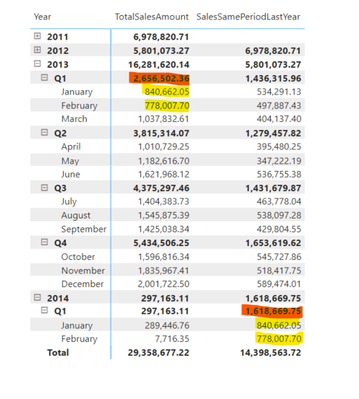cancel
Showing results for
Did you mean:New Member

## SAMEPERIODLASTYEAR() sums last quarter instead of showing the previous one

Hello,

I'm wondering how to force SAMEPERIODLASTYEAR() not to sum figures from the last available quarter taking into account that the quarter is not complete yet.

The example is below. The function correctly takes figures from January and February 2013, shows them near January and February 2014, but when it comes to the quarter result, instead of seeing figures from Q1 2013 (2,656,502.36) I got a simple sum of January&February 2014 which equals 1,618,669.75.

How to get 2,656,502.36 in the second column instead of 1,618,669.75?

Answers to the most typical questions:

1. I'm using a table that is marked as Date Table.

2. All connections between tables are established.

3. There are no dates after the 4th of February in both fact and dimension tables.1 ACCEPTED SOLUTIONNew Member

It turned out to be much easier. The date table should contain all dates related to the current year, even if the year hasn't ended yet. Then the calculations are correct.

3 REPLIES 3New Member

It turned out to be much easier. The date table should contain all dates related to the current year, even if the year hasn't ended yet. Then the calculations are correct.New Member

Hello @amitchandak

I'm currently using one SAMEPERIODLASTYEAR() formula that is applied to all time periods:

SalesSamePeriodLastYear = CALCULATE(SUM(fact_InternetSales[SalesAmount]), SAMEPERIODLASTYEAR(dim_Date[Date]))

How do I calculate subtotals separately?Super User

@AlinaP92 , You can use last year same qtr in sub total using isinscope

If(isinscope(date[Qtr]) && not(isinscope(Date[Month] )) , [Last year Qtr sales], [Sales Sameperiod last year ])

example

Last year same QTD Sales = CALCULATE(SUM(Sales[Sales Amount]),DATESQTD(dateadd('Date'[Date],-1,Year)))
Last year same QTR full  Sales = CALCULATE(SUM(Sales[Sales Amount]),DATESQTD(ENDOFQUARTER(dateadd('Date'[Date],-1,Year))))